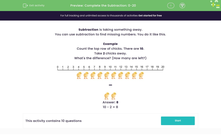# Complete the Subtraction: Numbers up to 20

In this worksheet, students will use subtraction to solve missing number problems.Key stage:  KS 1

Curriculum topic:   Number: Addition and Subtraction

Curriculum subtopic:   Add and Subtract to 20

Difficulty level:#### Worksheet Overview

Subtraction is taking something away.

You can use subtraction to find missing numbers. You do it like this.

Example

Count the top row of chicks. There are 10.

Take 2 chicks away.

What's the difference? (How many are left?)subtract10 - 2 = 8

Start at the number 10 on the line and move two places to the left on the line.

You reach the number 8. So you can see that 10 - 2 = 8You always move to the left on a number line when you are subtracting a number.Let's have a go at some questions now.### What is EdPlace?

We're your National Curriculum aligned online education content provider helping each child succeed in English, maths and science from year 1 to GCSE. With an EdPlace account you’ll be able to track and measure progress, helping each child achieve their best. We build confidence and attainment by personalising each child’s learning at a level that suits them.

Get started